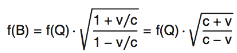# Download Modern Physics Equations and Formulas PDFTime Dilation
 t' = t √(1 − v2/c2)
Length Contraction
ℓ' = ℓ √(1 − v2/c2)
Relativistic Mass
 m' = m √(1 − v2/c2)
Relative Velocity
 u' = u + v 1 + uv/c2
Relativistic Energy
 E = mc2 √(1 − v2/c2)
Relativistic Momentum
 p = mv √(1 − v2/c2)
Energy-Momentum
E2 = p2 c2 + m02c4Mass-Energy
E = mc2
Relativistic Doppler EffectPhoton Energy
E = hf
Photoelectric Effect
Kmax = E − ϕ = h(ƒ − ƒ0)
Photon Momentum
 p = h λ
Schroedinger's Equation
 iℏ ∂ Ψ(r,t) = − ℏ2 ∇2Ψ(r,t) + V(r)Ψ(r,t) ∂t 2m
 Eψ(r) = − ℏ2 ∇2ψ(r) + V(r)ψ(r) 2m
Heisenberg's Uncertainty Principle
 Δpx Δx = ℏ 2
 ΔE Δt = ℏ 2
Rydberg Equation
 1 = −R∞ ⎛⎝ 1 − 1 ⎞⎠ λ n2 n02
Half Life
N = N02tMost popular math formulas with examples
Area formulas for all Shapes
Volume formulas for all Shapes
Rule of Surds
Law of Indices
Fourier Series formula
More >>Physics Equation with examples
Mechanics Equation
Electricity & Magnetism
Modern Physics Equation
Thermal Physics Equation
Waves Optics Equations
More >>